# Angle relationships#### Everything You Need in One Place

Homework problems? Exam preparation? Trying to grasp a concept or just brushing up the basics? Our extensive help & practice library have got you covered.#### Learn and Practice With Ease

Our proven video lessons ease you through problems quickly, and you get tonnes of friendly practice on questions that trip students up on tests and finals.#### Instant and Unlimited Help

Our personalized learning platform enables you to instantly find the exact walkthrough to your specific type of question. Activate unlimited help now!

##### Intros
###### Lessons
1. Introduction to Supplementary, Complementary, Vertical, and Adjacent Angles
2. What are supplementary angles?
3. What are complementary angles?
4. What are vertical angles?
##### Examples
###### Lessons
1. Application of Supplementary Angles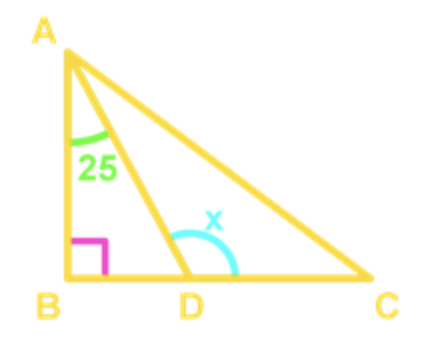Find the value of $x$.

1. Complementary Angles on Polygons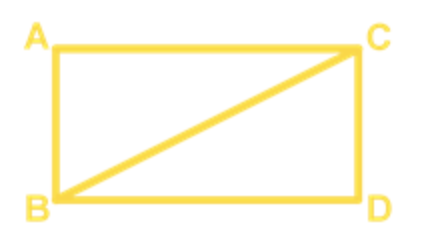Which of the following pair(s) is/are complementary angles?

1. $\angle ABD$ and $\angle ACD$
2. $\angle ABC$ and $\angle CBD$
3. $\angle ACB$ and $\angle CBD$
2.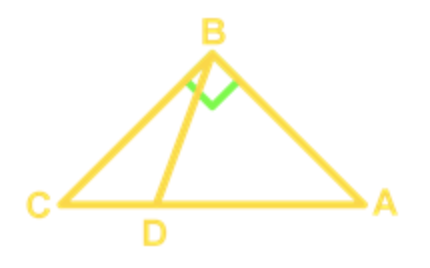Which of the following pair(s) is/are complementary angles?

1. $\angle CDB$ and $\angle ADB$
2. $\angle ABD$ and $\angle CBD$
3. $\angle BCD$ and $\angle BAD$
3. Application of Complementary Angles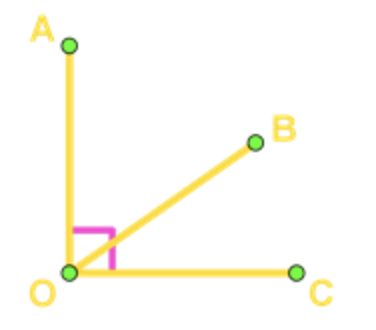If $\angle AOB=-9x+29$ and $\angle BOC=2x+82$, find the value of $x$.

1. Proving Vertical Angles Using Supplementary Angles

Use the idea of supplementary angles to prove for vertical angles.

1. Application of Vertical Angles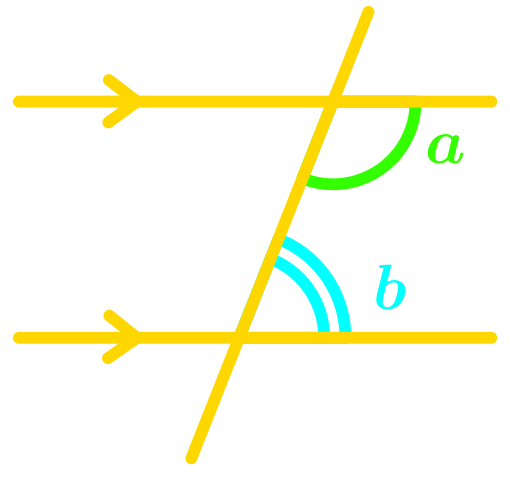Given that $\angle m=$43°, find the remaining angles in the diagram.

1.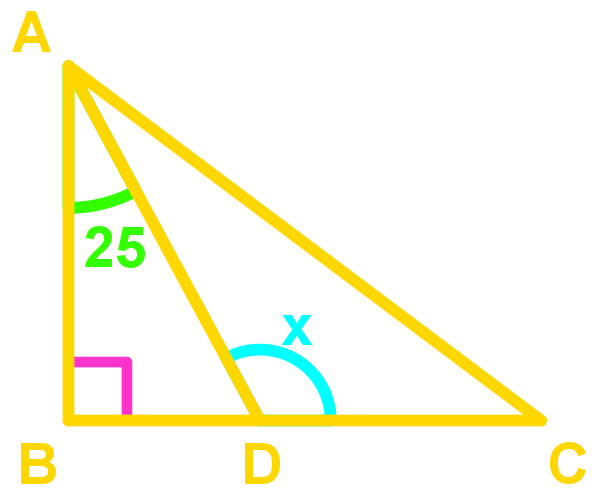Given that $\angle AOC$=125° and $\angle DOE$=35°.

1. Find $\angle BOC.$
2. Find $\angle EOB.$
2.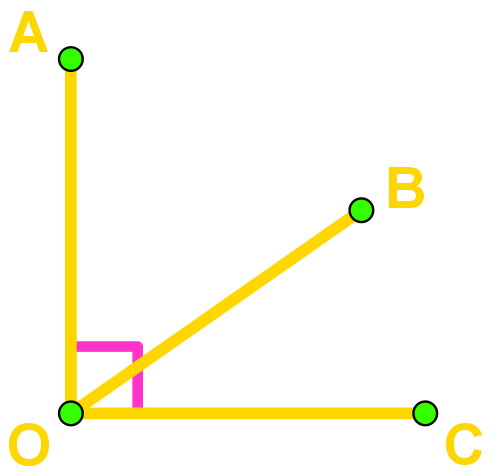If $\angle AOD$=3x+14 and $\angle BOC$=7x-18, find the value of x.

What of the following is/are adjacent angles?

1.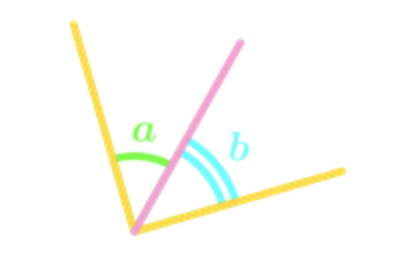2.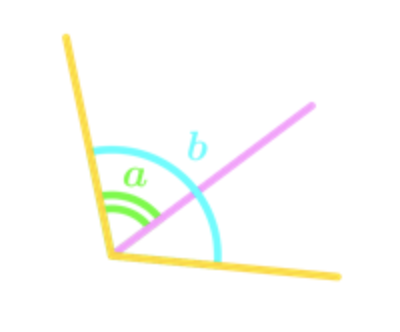3.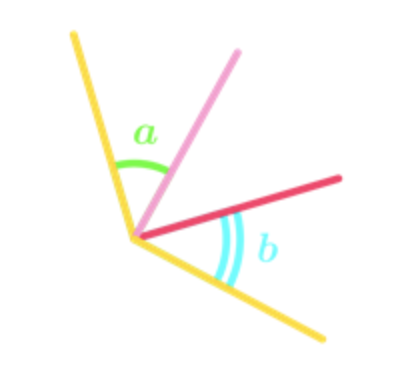4.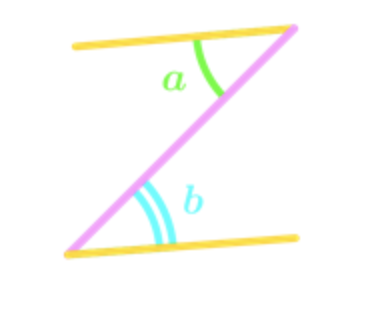2. Finding Adjacent Angles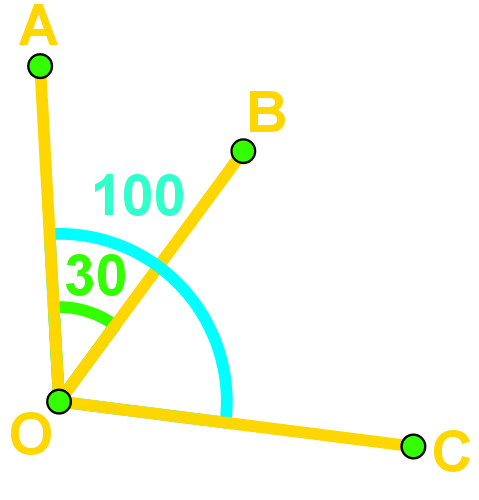$\angle AOB$=30°, $\angle AOC$=100°.

1. Are $\angle AOB$ and $\angle AOC$ adjacent?
2. Find an angle that is adjacent to $\angle AOB$.
###### Topic Notes
In this lesson, we will learn:
• Application of Supplementary Angles
• Complementary Angles on Polygons
• Application of Complementary Angles
• Proving Vertical Angles Using Supplementary Angles
• Application of Vertical Angles